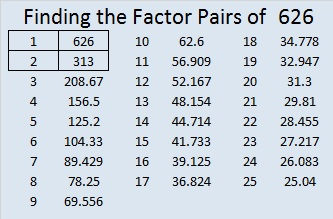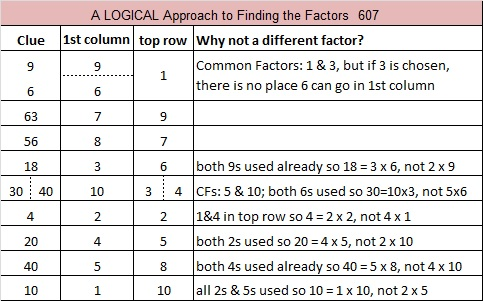# 626 Semordnilaps, Palindromes and Level 4

626 is the hypotenuse of the Pythagorean triple 50-624-626. What is the greatest common factor of those three numbers?

Today my son posted on facebook, “A word that when spelled backwards spells a different word is called a Semordnilap.” That’s a word I hadn’t heard before, and it also applies to phrases and sentences that form different phrases or sentences when read backwards.

“Semordnilap” is a semordnilap for the word “palindromes” which are words, phrases, sentences, and numbers that read the same forward and backward.

Palindrome sentences are sometimes made with words that are semordnilaps: was, saw, live, evil, on, no, desserts, stressed, stop, pots, tops, spot, diaper, repaid.

626 is a number that is a palindrome in several different bases:

• 10001 in base 5; note that (5^4) + 1 = 626.
• 626 in base 10
• 272 in base 16; note that 2(16^2) + 7(16) + 2 = 626
• 1DI in base 19, if “1” and “I” look the same, and too often they do. Note that 1(19^2) + 13(19) + 18 = 626
• 101 in base 25; note that (25^2) + 1 = 626
• 11 in base 625; note that 626 + 1 = 626

I guess we could say that in all other bases 626 is a semordnilap.

All of 626’s factors are palindromes, too.Print the puzzles or type the solution on this excel file: 12 Factors 2015-09-21

—————————————————————————————————

• 626 is a composite number.
• Prime factorization: 626 = 2 x 313
• The exponents in the prime factorization are 1 and 1. Adding one to each and multiplying we get (1 + 1)(1 + 1) = 2 x 2 = 4. Therefore 626 has exactly 4 factors.
• Factors of 626: 1, 2, 313, 626
• Factor pairs: 626 = 1 x 626 or 2 x 313
• 626 has no square factors that allow its square root to be simplified. √626 ≈ 25.019992.—————————————————————————————————# 607 and Level 6

607 is the sum of the three prime numbers from 197 to 211.Print the puzzles or type the solution on this excel file: 10 Factors 2015-09-01

—————————————————————————————————

• 607 is a prime number.
• Prime factorization: 607 is prime.
• The exponent of prime number 607 is 1. Adding 1 to that exponent we get (1 + 1) = 2. Therefore 607 has exactly 2 factors.
• Factors of 607: 1, 607
• Factor pairs: 607 = 1 x 607
• 607 has no square factors that allow its square root to be simplified. √607 ≈ 24.637How do we know that 607 is a prime number? If 607 were not a prime number, then it would be divisible by at least one prime number less than or equal to √607 ≈ 24.6. Since 607 cannot be divided evenly by 2, 3, 5, 7, 11, 13, 17, 19, or 23, we know that 607 is a prime number.

—————————————————————————————————